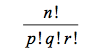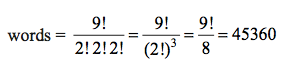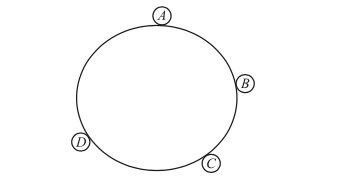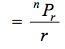In this section, the following topics are discussed with examples

• Counting formula for linear permutations
• With Repetition
• Examples
• Circular Permutations
• Examples

### Counting Formula for Linear Permutations## The permutations and combinations are endless. It is like a game of three dimensional chess.

Sherry Bebitch Jeffe

#### Example 1:

Find the number of ways in which four persons can sit on six chairs.

Solution:

6P4 = (6 x 5 x 4 x 3 x 2 x 1) /(2 x 1) = 360

## With Repetition

1. Number of permutations of things taken all at a time, if out of things are alike of one kind, are alike of second kind, are alike of a third kind and the rest – (r) are all different is2. Number of permutations of different things taken at a time when each thing may be repeated any number of times is n r.

#### Example 2

Find the number of words that can be formed out of the letters of the word COMMITTEE taken all at a time.

Solution:

There are 9 letters in the given word in which two T’s, two M’s and two E’s are identical. Hence the required number of## Circular Permutations

#### Arrangement Around a Circular Table

In circular arrangements, there is no concept of starting point (i.e. starting point is not defined). Hence number of circular permutations of different things taken all at a time is

(– 1)! if clockwise and anti-clockwise order are taken as different.In the case of four persons ABand sitting around a circular table, then the two arrangements ABCD (in clock- wise direction) and ADCB (the same order but in anti- clockwise direction) are different.
Hence the number of arrangements (or ways) in which four different persons can sit around a circular table = (4 – 1)! = 3! = 6.

#### Number of Circular Permutations of n Different Things Taken r at a Time

Case I: If clockwise and anti-clockwise orders are taken as different, then the required number of circular permutationsCase II: If clockwise and anti-clockwise orders are taken as same, then the required number of circular permutations =  nPr / 2r

#### Example 3

In how many ways can 5 boys and 5 girls be seated at a round table so that no two girls may be together ?

Solution:

Leaving one seat vacant between two boys, 5 boys may be seated in 4! ways. Then at remaining 5 seats, 5 girls can sit in 5! ways. Hence the required number

= 4! × 5! = 2880 ways.